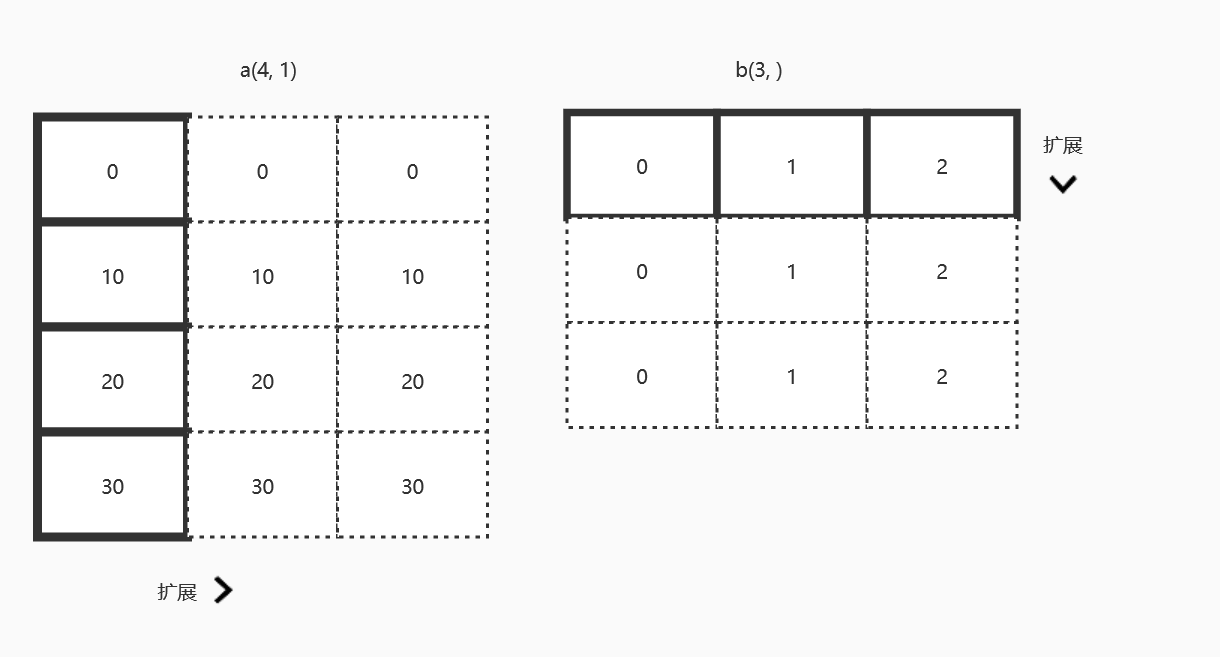# NumPy

## 初识 NumPy

NumPy 的主要对象是同质多维数组，也就是在一个元素（通常是数字）表中，元素的类型都是相同的。其中可以通过正整数的元组来对元素进行索引。

NumPy 的数组称为 ndarray， 别名为 array。numpy。array 与 Python 标准库里的 array.array 不一样，标准库只能处理一维数组并且功能相对较少。

ndarray 对象属性

T 转置
size 数组的元素的个数
itemsize 每个元素的大小
dtype 数组元素的数据类型对象
ndim 数组的轴数量
shape 数组的维度
Flat 返回数组的一维迭代器
imag 返回数组的虚部
real 返回数组的实部
nbytes 数组中所有元素的字节长度

## NumPy 数组类型

• int8, int16, int32, int64, uint8, uint16, uint32, uint64
• float16, float32, float64
• complex64, complex128
可以使用 dtype 来指定数据类型，也可以通过 astype()

>>>print(np.array(5, dtype=int32))

5

>>>print(np.array(5, astype(float)))

5.0

## NumPy 创建数组

• 可以用 numpy.array 来将列表或元组转换为 ndarray 数组，可以通过 order= 来指定顺序

• 使用 arange()，和 range() 基本一样，可以指定 dtype 来设置返回的 ndarray 的类型

• linspace() 生成等差数列

numpy.linspace(start, stop, num=50, endpoint=True, retstep=False, dtype=None)

endpoint 表示最后一个样本在不在序列内， retstep 为 True 时会返回间距

• 使用 logspace 生成等比数列

numpy.logspace(start, stop, num=50, endpoint=True, base=10.0, dtype=None)

base 表示基底，取对数时 log 的下标

• ones, zeros 分别创建一个指定形状的全1数组和全0数组

## 矩阵的合并与分割

### 矩阵的合并

hstack() 左右合并, vstack() 上下合并

## 矩阵运算与线性代数

### 求范数

np.linalg.norm(x, ord=None, axis=None, keepdims=False)

• x 表示要度量的向量
• ord 处理范数的种类
ord= 描述 公式
ord=2 二范数 $\sqrt{ \sum_{i=1}^{n}x_{i}^{2}}$
ord=1 一范数 $\sum_{i=1}^{n} \left| x_{i}\right|$
ord=np.inf 无穷范数 $max(\left| {x_{i}} \right|)$

### 最小二乘求线性函数

numpy.linalg.lstsq(array_A, array_B)

### 奇异值分解

U, Sigma, V = np.linalg.svd(D)

## NumPy 的广播机制

1. 让所有的输入数组都向维度最长的数组看齐，维度不足的部分通过在前面加1补齐
2. 输出数组的维度是输入数组维度的各个轴上的最大值
3. 如果输入数组的某个轴和输出数组的对应轴的长度相同或者都为1时，这个数组能够用来计算，否则出错
4. 如果输入数组的某个轴的长度为1时，沿着此轴运算时都用此轴上的第一组值
• 如果任何一个维度是1，那么另一个不为1的维度将被用作最终结果的维度。也就是说为1的维度将延展到与另一个维度匹配。## numpy 统计函数

• 用的时候可以再查

### 极差

• numpy.ptp(ndarray[, axis=]) 可以用 axis 指定行或列轴, axis=0 为列轴

## NumPy 排序，搜索

• numpy.sort

可以指定 axis = None, 0, 1 来指定排序方法， None 会将结果折叠为一维数组，0会按列处理， 默认为1，按行处理

• numpy.argsort
对 a 排序，a 不变，返回一个排序后的索引，其余和 sort 相同

• numpy.lexsort
支持对数组按指定行或列的顺序来排序，是间接排序

• argmax, nargmax
如果不指定 axis 则返回平坦化的下标，指定会按指定列或行轴来返回最值下标

Ctwo

2020-01-21

2020-10-25